# Surprise Drawing Machine

Choose.

That is the only instruction, along with three circles of different color, on the “menu” page, the default starting page of my drawing machine. My intention was to create a program which gives the users a sense of discovery and surprise.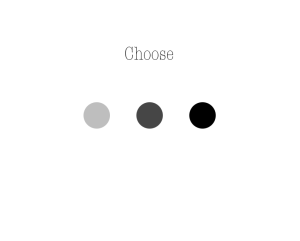This is why the initial screen presents the users with three options, but no instructions as to what any of their choices will lead to. This way, they can explore the machine step by step, function by function.

The first circle leads to a drawing machine which is set at automatic by default, and which uses a continuous line of circles of three repeating colors to create trippy images. The users can intervene in the process: a left mouse click changes the x direction, a right mouse click changes the y direction, and + and – signs control the size of the line. (Although these are not explained in the program, the functions can even be discovered by intuition.) Also, a menu bar is provided, with options, “Menu” (which leads back to the start page), “Clear” (which clears the image and generates a new random start point for the line), and “Auto Off” (which stops the line’s automated motion and gives control in the users’ hands, where drawing with the right mouse button means erase).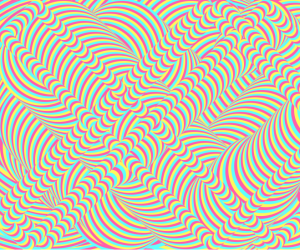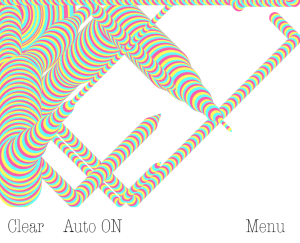//automatically generated images with user intervention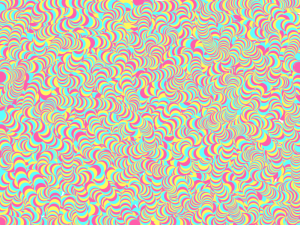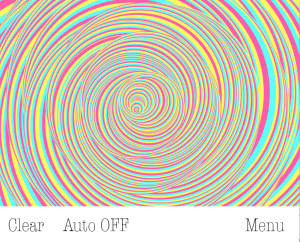//my favourite user-drawn images so far

The second function leads to a universal drawing tool, in which the users can control the color of the lines with the help of the toolbar provided on the right, and the rest of the characteristic in the same way as in the first function. If the users turn the auto mode on, the machine will draw an automated sequence of random shapes (different circles and rectangles). The color can still be controlled, and the machine can be paused by pressing “.” .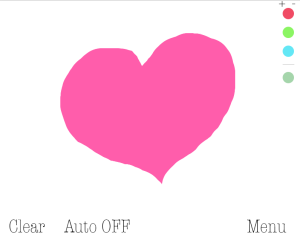// user-drawn image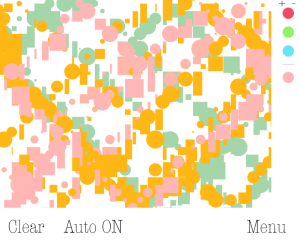// an automated image in very ugly colors

The third function is also set at auto by default. This function uses the same three colors as the first one, but this part of the machine generates a random pattern of “stars” (6 lines), the sizes of which can still be controlled. When auto mode is turned off, the machine chooses a random point and connects the location of the mouse with it whenever the mouse button is pressed.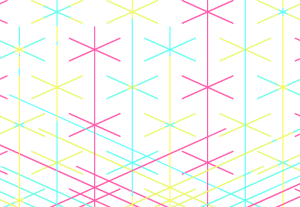//automated image in this function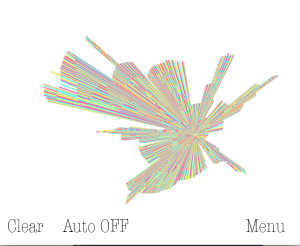// user-drawn image in this function

You can see my code below:

```PImage choose;

int red = 0;
int green =0;
int blue = 0;
float count = -1;
int function = 0;
int auto = 0;
int draw = 0;
float xSpeed = 4;
float ySpeed = 4;
float xPos;
float yPos;
float randXPos;
float randYPos;
float diam=30;
float rnd;
int stop = 0;

void setup()
{
size(800, 650);
background(255);
}

void draw()
{
randXPos = random(0, width);
randYPos = random(0, height-100);

if (count == -1)
{
background(255);
noStroke();
ellipseMode(CORNER);
image(choose, 307, 100);
fill(190);
ellipse(225, 275, 70, 70);
fill(70);
ellipse(365, 275, 70, 70);
fill(0);
ellipse(505, 275, 70, 70);
}

else if (function == 1)
{
trippy();
count+=1;
if (count==3)
{count=0;}
}

else if (function == 2){create();}

else if (function == 3)
{
stars();
count+=1;
if (count==3)
{count=0;}
}

}

void mouseClicked()
{
if (225 < mouseX && 295 > mouseX && 275 < mouseY && 345 > mouseY && count == -1)
{
function = 1;
count = 0;
print("functionone");
background(255);
xPos = randXPos;
yPos = randYPos;
xSpeed = 4;
ySpeed = 4;
stop = 0;
diam = 30;
}

if (365 < mouseX && 435 > mouseX && 275 < mouseY && 345 > mouseY && count == -1)
{
function = 2;
count = 0;
print("functiontwo");
background(255);
xSpeed=20;
ySpeed=20;
auto = 1;
xPos = random(0, 720);
yPos = random(0, 550);
stop = 0;
diam = 30;
}

if (505 < mouseX && 575 > mouseX && 275 < mouseY && 345 > mouseY  && count == -1)
{
function = 3;
count = 0;
print("functionthree");
background(255);
xPos = randXPos;
yPos = randYPos;
xSpeed=100;
ySpeed=100;
stop = 0;
diam = 30;

}

if (655 < mouseX && 550 < mouseY) //back to menu
{
count = -1;
}

if (117 > mouseX && 550 < mouseY) // clear
{
background(255);
stop = 0;

if (function == 1 || function == 3){
xPos = randXPos;
yPos = randYPos;}

if (function == 1)
{xSpeed = 4; ySpeed = 4;}

if (function == 2){
xPos = random(0, 720);
yPos = random(0, 550);}
}

if (150 < mouseX && 340 > mouseX && 550 < mouseY) //auto on/off
{
stop = 0;
if (auto == 0)
{auto = 1;}
else if (auto == 1)
{auto = 0;}
background(255);
xPos = randXPos;
yPos = randYPos;
}

if( 740 < mouseX && 755 > mouseX && 120 < mouseY && 150 > mouseY && function == 2 && blue < 255)
{blue+=10;
println(blue);}
if( 775 < mouseX && 790 > mouseX && 120 < mouseY && 150 > mouseY && function == 2 && blue >=0)
{blue-=10;
println(blue);}
if( 740 < mouseX && 755 > mouseX && 70 < mouseY && 100 > mouseY && function == 2 && green < 255)
{green+=10;
println(green);}
if( 775 < mouseX && 790 > mouseX && 70 < mouseY && 100 > mouseY && function == 2 && green >= 0)
{green-=10;
println(green);}
if( 740 < mouseX && 755 > mouseX && 20 < mouseY && 50 > mouseY && function == 2 && red < 255)
{red+=10;
println(red);}
if( 775 < mouseX && 790 > mouseX && 20 < mouseY && 50 > mouseY && function == 2 && red >= 0)
{red-=10;
println(red);}

}

void mousePressed()
{draw = 1;}

void mouseReleased()
{draw = 0;}

{
if (auto==0)
{
}

if (auto==1)
{
}
}

void trippy()
{
if (count==0)
{ red = 255;
green = 93;
blue = 171;
}

if (count==1)
{red = 255;
green = 247;
blue = 93;
}

if (count==2)
{ red = 93;
green = 255;
blue = 255;
}
fill(red, green, blue);
ellipseMode(CENTER);

if(keyPressed)
{
if(key=='+' && diam <800)
{diam+= 5;}
if(key=='-' && diam >0)
{diam+= -5;}
}

if (auto==0 && stop ==0)
{
ellipse(xPos, yPos, diam, diam);
xPos+= xSpeed;
yPos += ySpeed;

if(xPos > width-diam/2 || xPos < diam/2){
xSpeed = xSpeed* -1;
}
if(yPos > 550-diam/2 || yPos < diam/2){
ySpeed = ySpeed* -1;
}
if (mousePressed && (mouseButton == RIGHT))
{xSpeed = xSpeed* -1;}
if (mousePressed && (mouseButton == LEFT))
{ySpeed = ySpeed* -1;}
}

if(keyPressed)
{if(key=='.')
{if (stop==0){stop = 1;}
else if (stop==1){stop=0;}}
}

if (auto==1)
{
if(draw == 1){
noStroke();
if (mouseY < 550)
{
ellipse(mouseX, mouseY, diam, diam);
}
}
}
}

void create()
{
fill(255);
rectMode(CORNER);
rect(720,0,800,550);
fill(240, 75, 100);
ellipseMode(CORNER);
ellipse(750, 20, 30, 30);
fill(139, 245, 99);
ellipse(750, 70, 30, 30);
fill(99,231,245);
ellipse(750, 120, 30, 30);
stroke(180);
line(750, 170, 780, 170);
noStroke();
fill(255);
fill(red, green, blue);
ellipse(750, 190, 30, 30);

ellipseMode(CENTER);
if(keyPressed)
{
if(key=='+' && diam <800)
{diam+= 5;}
if(key=='-' && diam >0)
{diam+= -5;}
}
if (auto == 1 && draw == 1 && mouseX <720 && mouseY <550)
{

fill(red, green, blue);
if(mouseButton==RIGHT)
{fill(255);}

ellipse(mouseX, mouseY, diam, diam);
}

if (auto == 0)
{
if(stop==0)
{
rnd= random(0,2);
println(rnd);
diam = random(5,50);
if(rnd<.75)
{ellipse(xPos, yPos, diam, diam);
println("circle");}
else if(.75<= rnd && rnd<1.25)
{rect(xPos, yPos, diam, diam);
println("square");}
else if(1.25<=rnd)
{rect(xPos, yPos, diam/2, diam+15);
println("rect");}
xPos+= xSpeed;
yPos+=ySpeed;
if(xPos > width-80-diam/2 || xPos < diam/2){
xSpeed = xSpeed* -1;
}
if(yPos > 550-diam/2 || yPos < diam/2){
ySpeed = ySpeed* -1;
}
}

if(keyPressed)
{if(key=='.')
{if (stop==0){stop = 1;}
else if (stop==1){stop=0;}}
}
}
}

void stars()
{
if(keyPressed)
{
if(key=='+' && diam <800)
{diam+= 5;}
if(key=='-' && diam >0)
{diam+= -5;}
}

if(keyPressed)
{if(key=='.')
{if (stop==0){stop = 1;}
else if (stop==1){stop=0;}}
}

strokeWeight(3);
if (count==0)
{ red = 255;
green = 93;
blue = 171;
}

if (count==1)
{red = 255;
green = 247;
blue = 93;
}

if (count==2)
{ red = 93;
green = 255;
blue = 255;
}
stroke(red, green, blue);

if (auto==0 && stop==0)
{

println(xPos);
println(yPos);

line(xPos, (yPos), (xPos+(sqrt(5)*diam)), (yPos+diam));
line(xPos, (yPos), (xPos-(sqrt(5)*diam)), (yPos-diam));
line(xPos, (yPos), (xPos+(sqrt(5)*diam)), (yPos-diam));
line(xPos, (yPos), (xPos-(sqrt(5)*diam)), (yPos+diam));
line(xPos, yPos, xPos, yPos+2*diam);
line(xPos, yPos, xPos, yPos-2*diam);
xPos+= xSpeed;
yPos+=ySpeed;
if(xPos > width || xPos < 0){
xSpeed = xSpeed* -1;
}
if(yPos > 550 || yPos < 0){
ySpeed = ySpeed* -1;
}
if (mousePressed && (mouseButton == RIGHT))
{xSpeed = xSpeed* -1;}
if (mousePressed && (mouseButton == LEFT))
{ySpeed = ySpeed* -1;}

}

if (auto == 1 && draw == 1 && mouseY<550)
{
line(xPos, yPos, mouseX, mouseY);
}

}```

Note: for the images to load, they have to be loaded to a subfolder named “data” in the directory of the .pde file